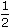# Aptitude - Boats and Streams

### Exercise :: Boats and Streams - General Questions

6.

A boat running downstream covers a distance of 16 km in 2 hours while for covering the same distance upstream, it takes 4 hours. What is the speed of the boat in still water?

 A. 4 km/hr B. 6 km/hr C. 8 km/hr D. Data inadequate

Explanation:

 Rate downstream =16kmph = 8 kmph. 2

 Rate upstream =16kmph = 4 kmph. 4Speed in still water = 1 (8 + 4) kmph = 6 kmph. 2

Video Explanation: https://youtu.be/3D8uAfzz3l0

7.

The speed of a boat in still water in 15 km/hr and the rate of current is 3 km/hr. The distance travelled downstream in 12 minutes is:

 A. 1.2 km B. 1.8 km C. 2.4 km D. 3.6 km

Explanation:

Speed downstream = (15 + 3) kmph = 18 kmph.

 Distance travelled =18 x 12km = 3.6 km. 60

Video Explanation: https://youtu.be/IDOwWUeOldQ

8.

A boat takes 90 minutes less to travel 36 miles downstream than to travel the same distance upstream. If the speed of the boat in still water is 10 mph, the speed of the stream is:

 A. 2 mph B. 2.5 mph C. 3 mph D. 4 mph

Explanation:

Let the speed of the stream x mph. Then,

Speed downstream = (10 + x) mph,

Speed upstream = (10 - x) mph.36 - 36 = 90 (10 - x) (10 + x) 6072x x 60 = 90 (100 - x2)x2 + 48x - 100 = 0(x+ 50)(x - 2) = 0x = 2 mph.

9.

A man can row at 5 kmph in still water. If the velocity of current is 1 kmph and it takes him 1 hour to row to a place and come back, how far is the place?

 A. 2.4 km B. 2.5 km C. 3 km D. 3.6 km

Explanation:

Speed downstream = (5 + 1) kmph = 6 kmph.

Speed upstream = (5 - 1) kmph = 4 kmph.

Let the required distance be x km.

 Then, x + x = 1 6 42x + 3x = 125x = 12x = 2.4 km.

10.

A boat covers a certain distance downstream in 1 hour, while it comes back in 1hours. If the speed of the stream be 3 kmph, what is the speed of the boat in still water?

 A. 12 kmph B. 13 kmph C. 14 kmph D. 15 kmph E. None of these

Explanation:

Let the speed of the boat in still water be x kmph. Then,

Speed downstream = (x + 3) kmph,

Speed upstream = (x - 3) kmph.(x + 3) x 1 = (x - 3) x 3 22x + 6 = 3x - 9x = 15 kmph.